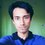# Proof Problem Of The Day - The Chess Tournament!

In a chess tournament of $$n$$ players, everyone played against everyone. Luckily enough, there were no draws. Prove that it is possible to label the players as $$A_1$$, $$A_2$$, $$A_3$$, $$\cdots$$ $$A_n$$ such that $$A_i$$ won against $$A_{i+1}$$ for $$i \in \{1, 2, 3, \cdots n-1\}$$

Click here to see all the problems posted so far. Keep checking everyday!Note by Mursalin Habib
6 years, 10 months ago

This discussion board is a place to discuss our Daily Challenges and the math and science related to those challenges. Explanations are more than just a solution — they should explain the steps and thinking strategies that you used to obtain the solution. Comments should further the discussion of math and science.

When posting on Brilliant:

• Use the emojis to react to an explanation, whether you're congratulating a job well done , or just really confused .
• Ask specific questions about the challenge or the steps in somebody's explanation. Well-posed questions can add a lot to the discussion, but posting "I don't understand!" doesn't help anyone.
• Try to contribute something new to the discussion, whether it is an extension, generalization or other idea related to the challenge.
• Stay on topic — we're all here to learn more about math and science, not to hear about your favorite get-rich-quick scheme or current world events.

MarkdownAppears as
*italics* or _italics_ italics
**bold** or __bold__ bold
- bulleted- list
• bulleted
• list
1. numbered2. list
1. numbered
2. list
Note: you must add a full line of space before and after lists for them to show up correctly
paragraph 1paragraph 2

paragraph 1

paragraph 2

[example link](https://brilliant.org)example link
> This is a quote
This is a quote
    # I indented these lines
# 4 spaces, and now they show
# up as a code block.

print "hello world"
# I indented these lines
# 4 spaces, and now they show
# up as a code block.

print "hello world"
MathAppears as
Remember to wrap math in $$ ... $$ or $ ... $ to ensure proper formatting.
2 \times 3 $2 \times 3$
2^{34} $2^{34}$
a_{i-1} $a_{i-1}$
\frac{2}{3} $\frac{2}{3}$
\sqrt{2} $\sqrt{2}$
\sum_{i=1}^3 $\sum_{i=1}^3$
\sin \theta $\sin \theta$
\boxed{123} $\boxed{123}$

## Comments

Sort by:

Top Newest

BdMO 2014 - Sec 9

Consider the complete directed graph with $n$ vertices where $A\longrightarrow B$ denotes $A$ won against $B$. Then the problem can be rephrased in the language of Graph Theory as a well-known statement: Prove that every complete directed graph contains a Hamiltonian path.

We proceed by induction on $n$. Verify the trivial cases $n=1,2$. We assume that the statement is true for $n=k$ and consider the complete directed graph $G$ with $n=k+1$ vertices. Take a vertex $p$ of $G$. Now applying the inductive hypothesis, consider a Hamiltonian path $\{p_1\to p_2\to\cdot\cdot\cdot\to p_k\}$ in $G-\{p\}$ which clearly has $k$ vertices. Now let $m\in\{1,2,...,k\}$ be the minimal element such that the edge $p \longrightarrow p_m$ exists. Then

$\left\{p_1\to p_2\to \cdot\cdot\cdot\to p_{m-1}\to p\to p_m \to \cdot\cdot\cdot\to p_k\right\}$

clearly is a Hamiltonian path. If such an $m$ doesn't exist, just append the edge $p_k\longrightarrow p$. This completes the induction. $\square$

- 6 years, 10 months ago

Log in to reply

There's a shorter approach that uses strong induction.

Assume that the claim is true for a tournament of any size $m\leq k$. For a tournament of $k+1$ players, consider any player (let's call him Nirjhor). Now divide the rest of the players into two sets; one that contains all the players Nirjhor has won against and another that contains all the players that contain all the players Nirjhor has lost against. Both of these sets have size $\leq k$.

I hope everyone can take it from here.

- 6 years, 10 months ago

Log in to reply

Great approach. But I don't see how this is shorter.

- 6 years, 10 months ago

Log in to reply

It isn't? It seemed shorter to me.

It certainly is much sleeker [to me] if not shorter.

- 6 years, 10 months ago

Log in to reply

I also think this one sleeker as Nirjhor's proof went a bit over my head! (No Offense)

- 6 years, 10 months ago

Log in to reply

Given 8 distinguishable rings ,find the number of possible S-ring arrangements on the four fingers(not the thumb) of one hand .(the order of the rings on each finger significant,but it is not required that each finger have a ring).

- 5 years, 11 months ago

Log in to reply

×

Problem Loading...

Note Loading...

Set Loading...